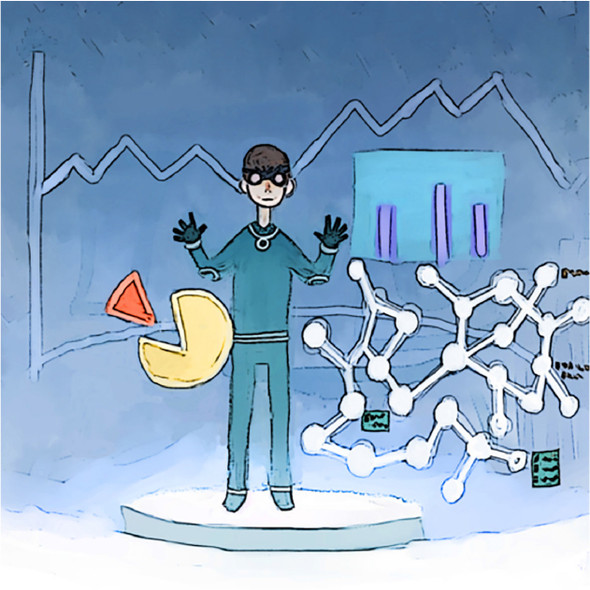# Chapter 3. Linear regression and beyondThis chapter covers

• Fitting a line to data points
• Fitting arbitrary curves to data points
• Testing performance of regression algorithms
• Applying regression to real-world data

Remember science courses back in high school? It might have been a while ago, or who knows—maybe you’re in high school now, starting your journey in machine learning early. Either way, whether you took biology, chemistry, or physics, a common technique to analyze data is to plot how changing one variable affects another.

Imagine plotting the correlation between rainfall frequency and agriculture production. You may observe that an increase in rainfall produces an increase in agriculture production rate. Fitting a line to these data points enables you to make predictions about the production rate under different rain conditions. If you discover the underlying function from a few data points, then that learned function empowers you to make predictions about the values of unseen data.

Regression is a study of how to best fit a curve to summarize your data. It’s one of the most powerful and well-studied types of supervised-learning algorithms. In regression, we try to understand the data points by discovering the curve that might have generated them. In doing so, we seek an explanation for why the given data is scattered the way it is. The best-fit curve gives us a model for explaining how the dataset might have been produced.